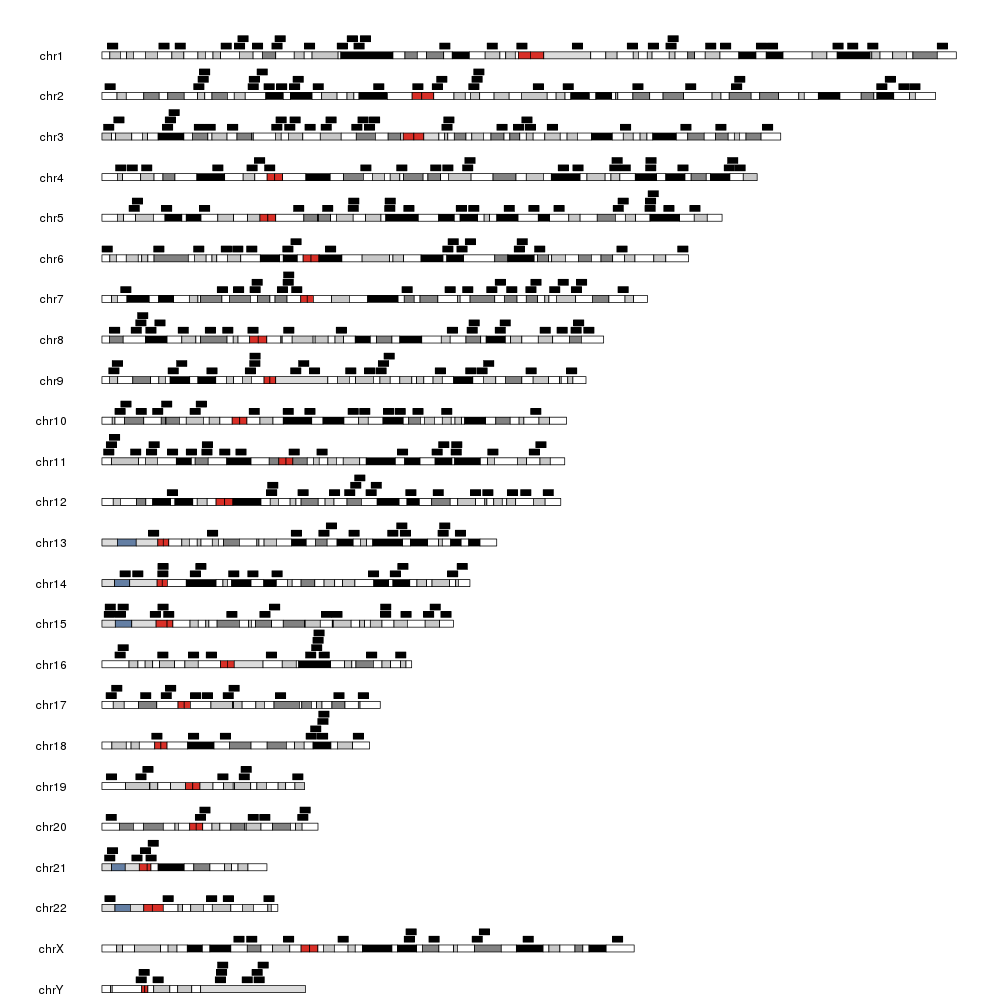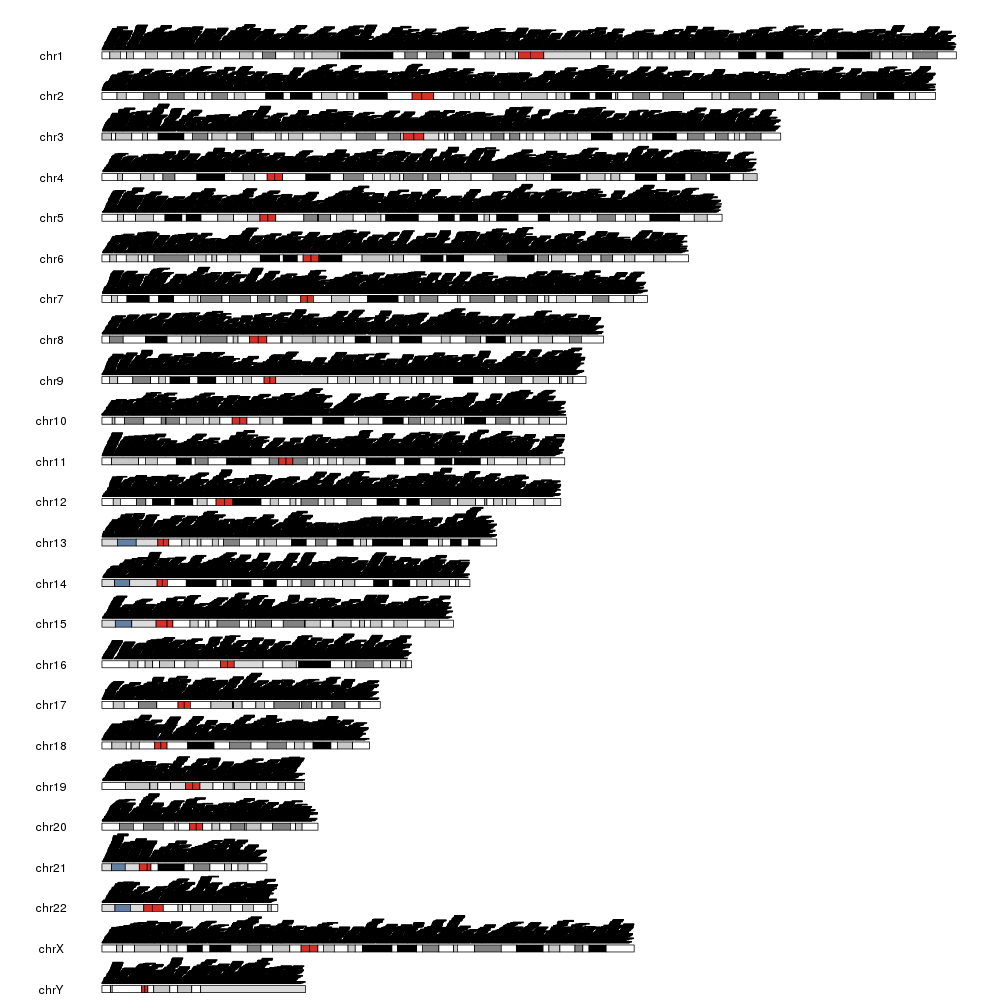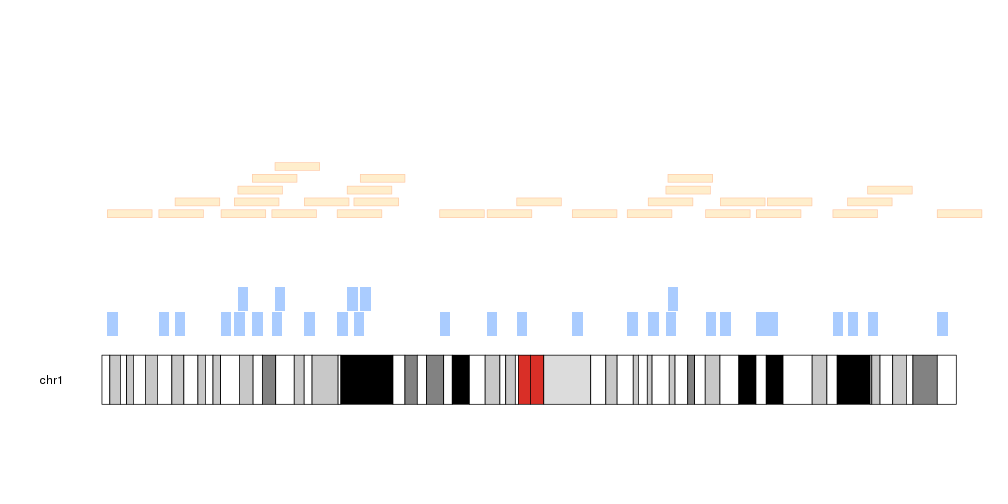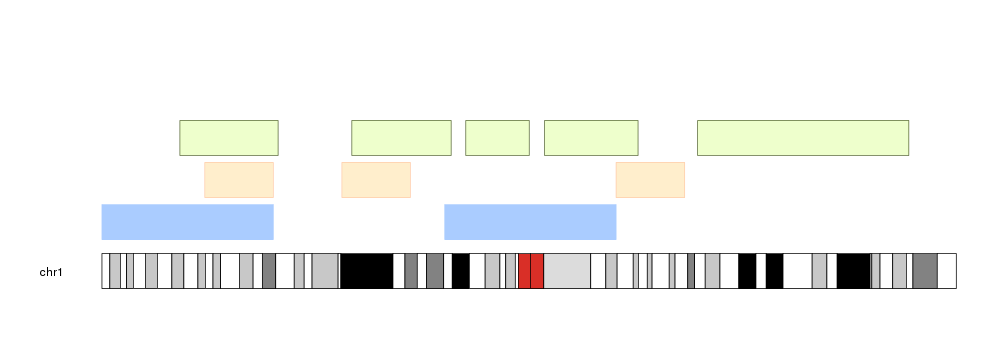## Plotting Genomic Regions

The function kpPlotRegions as its name suggest, can be used to plot regions along the genome. Regions are plotted as rectangles and the function includes an option (active by default) to plot overlapping regions as a stack. This function only accepts regions as GRanges objects and does not accept the chr, x0, x1, y0 and y2 parameters used by `kpRect`.

We will create a set of random regions using regioneR’s `createRandomRegions` function and plot them on the genome.

``````library(karyoploteR)
regions <- createRandomRegions(nregions=400, length.mean = 3e6, mask=NA, non.overlapping = FALSE)
kp <- plotKaryotype()
kpPlotRegions(kp, data=regions)
``````The height of the rectangles is adjusted so the tallest pile of regions fits in the data panel, making the regions flatter if needed. For example, plotting 40K regions produces a plot like this.

``````many.regions <- createRandomRegions(nregions=40000, length.mean = 3e6, mask=NA, non.overlapping = FALSE)
kp <- plotKaryotype()
kpPlotRegions(kp, data=many.regions)
``````It is possible to customize the appearance of the regions using the same parameters used for kpRect plus the additional parameters avoid.overlapping, num.layers and layer.margin to control the layering of the regions.

``````kp <- plotKaryotype(chromosomes="chr1")
kpPlotRegions(kp, data=regions, col="#AACCFF", layer.margin = 0.01, border=NA, r0=0, r1=0.5)
kpPlotRegions(kp, data=extendRegions(regions, extend.end = 10e6), col="#FFEECC", layer.margin = 0.05, border="#FFCCAA", r0=0.6, r1=1)
``````# Input Regions

As in most functions in karyoploteR, `kpPlotRegions` uses internally the `toGRanges`function from regioneR. This means that it’s possible to call `kpPlotRegions` with a bed-like file, even a remote one, a data.frame or even an array of characters defining the regions. You can find more information on the valid formats in regioneR’s vignette.

``````kp <- plotKaryotype(chromosomes="chr1")
kpPlotRegions(kp, data=c("chr1:1-50000000", "chr1:100e6-150e6"), col="#AACCFF", r0=0, r1=0.25)
df <- data.frame(chr=c("chr1", "chr1", "chr1"), start=c(30e6, 70e6, 150e6), end=c(50e6, 90e6, 170e6))
kpPlotRegions(kp, data=df, col="#FFEECC", border="#FFCCAA", r0=0.3, r1=0.55)
kpPlotRegions(kp, data="Tutorial/PlotRegions/regions.txt", col="#EEFFCC", border=darker("#EEFFCC"), r0=0.6, r1=0.85)
``````